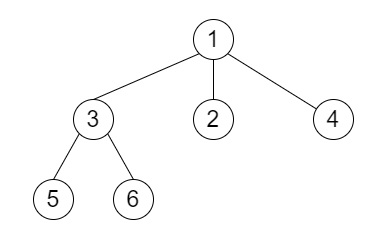# N-ary Tree Preorder Traversal in C++

Suppose we have one n-ary tree, we have to find the preorder traversal of its nodes.

So, if the input is likethen the output will be [1,3,5,6,2,4]

To solve this, we will follow these steps −

• Define an array ans

• Define a method called preorder(), this will take root

• if root is null, then −

• return empty list

• insert value of root at the end of ans

• for all child i in children array of root

• preorder(i)

• return ans

## Example

Let us see the following implementation to get a better understanding −

Live Demo

#include <bits/stdc++.h>
using namespace std;
void print_vector(vector<auto> v){
cout << "[";
for(int i = 0; i<v.size(); i++){
cout << v[i] << ", ";
}
cout << "]"<<endl;
}
class Node {
public:
int val;
vector<Node*> children;
Node() {}
Node(int _val) {
val = _val;
}
Node(int _val, vector<Node*> _children) {
val = _val;
children = _children;
}
};
class Solution {
public:
vector<int&g; ans;
vector<int> preorder(Node* root) {
if (!root)
return {};
ans.emplace_back(root->val);
for (auto i : root->children)
preorder(i);
return ans;
}
};
main(){
Solution ob;
Node *node5 = new Node(5), *node6 = new Node(6);
vector<Node*> child_of_3 = {node5, node6};
Node* node3 = new Node(3, child_of_3);
Node *node2 = new Node(2), *node4 = new Node(4);l
vector<Node*> child_of_1 = {node3, node2, node4};
Node *node1 = new Node(1, child_of_1);
print_vector(ob.preorder(node1));
}

## Input

Node *node5 = new Node(5), *node6 = new Node(6);
vector<Node*> child_of_3 = {node5, node6};
Node* node3 = new Node(3, child_of_3);
Node *node2 = new Node(2), *node4 = new Node(4);
vector<Node*> child_of_1 = {node3, node2, node4};
Node *node1 = new Node(1, child_of_1);

## Output

[1, 3, 5, 6, 2, 4, ]